﻿ GATE Exam marking scheme | ECE IES Reference books | IES and ESE reference books for Electronics and Communication Engineering
 Join  our Virtual Interactive Classroom NOW! Try out our Sample Test Watch our Sample Video Attend our  Orientation Programme Call: +91 9000018804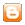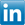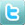Home | Institute | GATE | IES | Virtual Classroom | Courses | Faculty | Resources | Feedbacks | Contact Us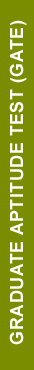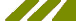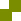GATE - MARKING SCHEME

GENERAL APTITUDE (GA) QUESTIONS:

In all papers, GA questions are of multiple choice type, and carry a total of 15 marks.  The GA section includes 5 questions carrying 1 mark each (sub-total 5 marks) and 5 questions carrying 2 marks each (sub-total 10 marks).

QUESTIONS PAPERS OTHER THAN GG, XE AND XL:

These papers would contain 25 questions carrying one mark each (sub-total 25 marks) and 30 questions carrying two marks each (sub-total 60 marks).  Out of these, two pairs of questions would be common data questions, and two pairs of questions would be linked answer questions.

In the ONLINE papers, the question paper will consist of  questions of multiple choice type and numerical answer type. For multiple choice type questions, each question will have four choices for the answer. For numerical answer type questions, each question will have a number as the answer and choices will not be given. The answer is to be entered using the mouse and a virtual keypad that will appear on the screen.

GG (GEOLOGY & GEOPHYSICS) PAPER:

Apart from the General Aptitude (GA) section, the GG question paper consists of two parts: Part A and Part B.

Part A is common for all candidates. Part A consists of 25 multiple choice questions carrying 1 mark each (sub-total 25 marks & some of these may be numerical questions).

Part B contains two sections:

• Section 1 (Geology)
• Section 2 (Geo-physics).

Each section  in Part B (Section 1 and Section 2) consists of 30 multiple choice questions carrying 2 marks each (sub-total 60 marks and some of these may be numerical questions). Out of these, two pairs of questions would be common data questions, and two pairs of questions would be linked answer questions.

Candidates will have to attempt questions in Part A and either Section 1 or Section 2 in Part B.

XE PAPER (ENGINEERING SCIENCES):

In XE paper, Engineering Mathematics section (Section A) is compulsory. This section contains 11 multiple choice questions carrying a total of 15 marks: 7 questions carrying 1 mark each (sub-total 7 marks), and 4 questions carrying 2 marks each (sub-total 8 marks). Some of the multiple choice questions may be replaced by numerical questions.

Each of the other sections of the XE paper (Sections B through G) contains 22 questions carrying a total of 35 marks9 questions carrying 1 mark each (sub-total 9 marks) and 13 questions carrying 2 marks each (sub-total 26 marks).  Out of the 2 mark questions, 2 pairs are common data questions and 1 pair is linked answer questions. Some of the multiple choice questions may be replaced by numerical questions.

XL PAPER (LIFE SCIENCES):

In XL paper,  Chemistry section (Section H) is compulsory. This section contains 15 multiple choice questions carrying a total of 25 marks: 5 questions carrying 1 mark each (sub-total 5 marks), and 10 questions carrying 2 marks each (sub-total 20 marks). Some of the multiple choice questions may be replaced by numerical questions.

Each of the other sections of the XL paper (Sections I through M) contains 20 multiple choice questions carrying a total of 30 marks: 10 questions carrying 1 mark each (sub-total 10 marks) and 10 questions carrying 2 marks each (sub-total 20 marks). Some of the multiple choice questions may be replaced by numerical questions.